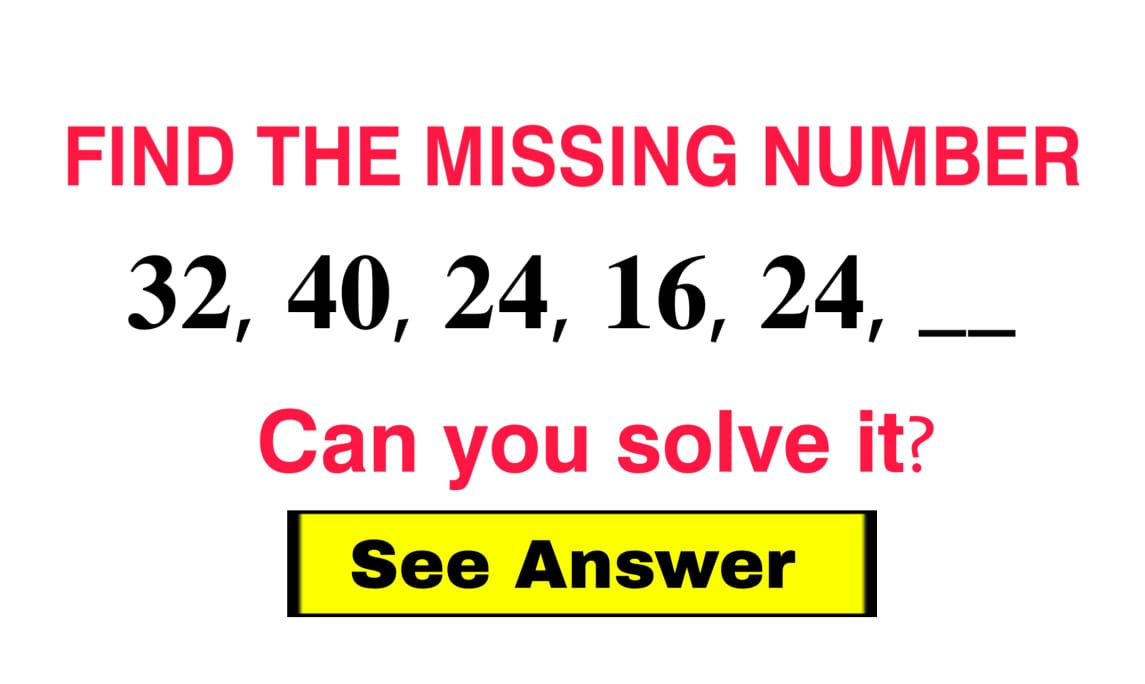# 10 Banking Aptitude Questions (Number Series) – Can you solve them? Try it!

Aptitude Questions are common in various competitive exams, including Banking exams.

In this article, we have given you 10 Free Banking aptitude Questions with detailed answers and explanations so that you can solve these and prepare for various online exam preparation, various interviews, Logical Reasoning Categories online tests and more.

## 10 NUMBER SERIES BANKING APTITUDE QUESTIONS

### (Q1-Q5) What will come in place of the blank (__) in each of the following series?

#### Q1. 2, 5, 9, 19, 37, __

OPTIONS

A. 73

B. 74

C. 75

D. 76

E. 77

ANS: C. 75

SOLUTION:

2*2+5=9
5*2+9=19
9*2+19=37
19*2+37=75
Therefore with this logic, the answer is 75.

#### Q2. 32, 40, 24, 16, 24, __

OPTIONS

A. 12

B. 18

C. 8

D. 32

E. 64

ANS: C. 8

SOLUTION:

As the 3rd number is less then 2nd,
Let's assume a numbers needs to be substracted or divided
Now, lets apply a logic,
32+8=40
32-8=24
Now lets apply the same logic again, 16+8=24
16-8=8
Therefore the ans is 8

#### Q3. 6, 7, 11, 20, 36, __

OPTIONS:

A. 66

B. 61

C. 68

D. 62

E. 64

ANS: B. 61

SOLUTION:

We can see,
7-6= 1 =1*1
11-7= 4 =2*2
20-11= 9 =3*3
36-20= 16 =4*4
Now lets apply the same logic again we get, 36+(5*5)=61
Therefore the ANS is 61

#### Q4. 7, 8, 18, 57, __, 1165

[SBI-PO 2017]

OPTIONS:

A. 212

B. 217

C. 232

D. 247

E. 275

ANS: C. 232

SOLUTION:

7x1+1=8
8x2+2=18
18x3+3=57
57x4+4=232
232x5+5=1165
Therefore the ANS is 232

#### Q5. 77, 85, 69, 101, 37, __

[SBI-PO 2017]

OPTIONS:

A. 105

B. 125

C. 145

D. 165

E. 185

ANS: D. 165

SOLUTION:

77+(8x1)=85
85-(8x2)=69
69+(8x4)=101
101-(8x8)=37
27+(8x16)=165
Therefore the ANS is 165

### (Q6-Q10) A number in the series is wrong. Find the wrong number.

#### Q6. 59, 60 ,61 ,64 ,79 ,185 ,1129

[SBI-PO 2018]

OPTIONS:

A. 185

B. 61

C. 60

D. 1129

E. 79

ANS: A. 185

SOLUTION:

The differences of the series is 1,3,15,106…
This can be formed on the basis like
1×1,1×3,3×5,15×7..so on
Hence the wrong no. is 185

#### Q7. 291, 147, 75, 39, 22, 12, 7.5

[SBI-PO 2018]

OPTIONS:

A. 39

B. 147

C. 22

D. 7.5

E. 291

ANS: C. 22

SOLUTION:

The series is
291−147=144
147−75=72
75−39=36
39−22=17 but this difference will be 18
So the wrong no. is 22

#### Q8. 3, 9, 19, 32, 51

OPTIONS:

A. 3

B. 9

C. 19

D. 32

E. 51

ANS: D. 32

SOLUTION:

The series is
2x1+1=3
2x4+1=9
2x9+1=19
2x16+1=33 but this is 32
So the wrong no. is 32

#### Q9. 26, 27, 34, 58, 106, 186, 306

[SBI-PO 2018]

OPTIONS:

A. 27

B. 34

C. 186

D. 106

E. 306

ANS: A. 27

SOLUTION:

The series is 26+(1x1)−1=26
26+(3x3)−1=34
34+(5x5)−1=58
58+(7x7)−1=106 and so on…
Hence wrong no. is 27

#### Q10. 1, 3, 9, 31, 129, 652

[SBI-PO 2018]

OPTIONS:

A. 1

B. 3

C. 652

D. 31

E. 129

ANS: C. 652

SOLUTION:

The series is
1×1+2=3
3×2+3=9
9×3+4=31
31×4+5=129
129×5+6=651
Hence the wrong no.is 652

How many could you solve? Comment them down below.